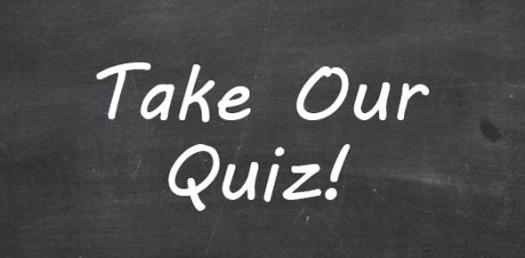# Systems And Linear Equations Assessment

11 Questions | Attempts: 693
ShareSettings.

• 1.
The sum of two factors of 48 is nineteen.  The larger factor, x, is one more than five times the smaller factor, y.  Which system of equations can be used to find the numbers?
• A.

X = 19 - y x = 5y + 1

• B.

X = 19 + y y = 5x + 1

• C.

X = y + 19 y = 5x - 1

• D.

X = y - 19 x = 5y + 1

• 2.
At September Fest, the first dance of the year, the Student Council charged \$3 for couples and \$2 for singles.  If 365 tickets were sold, and the total receipts were \$925, which pair of equations would determine the number of each ticket sold?
• A.

X - y = 365 3x + 2y = 925

• B.

X - y = 365 x + y = 925

• C.

X + y = 365 3x + 2y = 925

• D.

X + y = 925 2x + 3y = 365

• 3.
The length of a rectangle is twice its width, while the perimeter of the rectangle is 36 units.  Which system of equations can be used to find the length "L" and the width "W" of the rectangle?
• A.

2L = W 2L + 2W = 36

• B.

L = 2W 2L + 2W = 36

• C.

2L = W L + W = 18

• D.

L = 2W + 2 36 - W = L

• 4.
The drama department sold 300 tickets for their last show.  Adult tickets cost \$10 and student tickets cost \$5.  If they sold \$2750 worth of tickets, what is a reasonable conclusion that can be made about the number of tickets sold?
• A.

• B.

More adults than students bought tickets

• C.

More students than adults bought tickets

• D.

The same number of adults and students bought tickets

• 5.
Which of the following is closest to the solution to this system of linear equations?
• A.

(3.5, -2.125)

• B.

(3.25, -1.25)

• C.

(2.5, -0.33)

• D.

(2.66, -1.75)

• 6.
What is the solution to the system of equations represented by these tables?
• A.

(2,3)

• B.

(3,5)

• C.

(-1,1)

• D.

(5,11)

• 7.
• A.

(-1, -2)

• B.

(1, 2)

• C.

(2, 1)

• D.

(-1, 1)

• 8.
Dominica has a total of 22 coins in her pocket, all of them nickels and dimes.  If the value of the coins is \$1.50, then how many nickels does Dominica have?
• A.

8 nickels

• B.

10 nickels

• C.

12 nickels

• D.

14 nickels

• 9.
Dustin wants to buy 9 tickets to the circus.  The lower balcony tickets cost \$20.95 while the upper balcony tickets cost only \$12.50.  How many of each type of ticket did Dustin buy if he spent \$146.30?
• A.

2 lower balcony tickets, 7 upper balcony tickets

• B.

4 lower balcony tickets, 5 upper balcony tickets

• C.

5 lower balcony tickets, 4 upper balcony tickets

• D.

6 lower balcony tickets, 3 upper balcony tickets

• 10.
The graph of p = 500 and the graph of p = 25s - 100 intersect at the point (24, 500).  If "p" represents the profit and "s" represents sales, what does the intersection best represent?
• A.

The profit is always 500.

• B.

The sales are always 24.

• C.

When the sales are 500, the profit will be 24.

• D.

When the sales are 24, the profit will be 500.

• 11.
Bagels cost \$6.99 per dozen and muffins cost \$7.50 per dozen.  If Rita has at most \$75 to spend, which combination of bagels and muffins is not a reasonable purchase?
• A.

5 dozen bagels and 3 dozen muffins

• B.

2 dozen bagels and 8 dozen muffins

• C.

7 dozen bagels and 5 dozen muffins

• D.

4 dozen bagels and 4 dozen muffinsBack to top